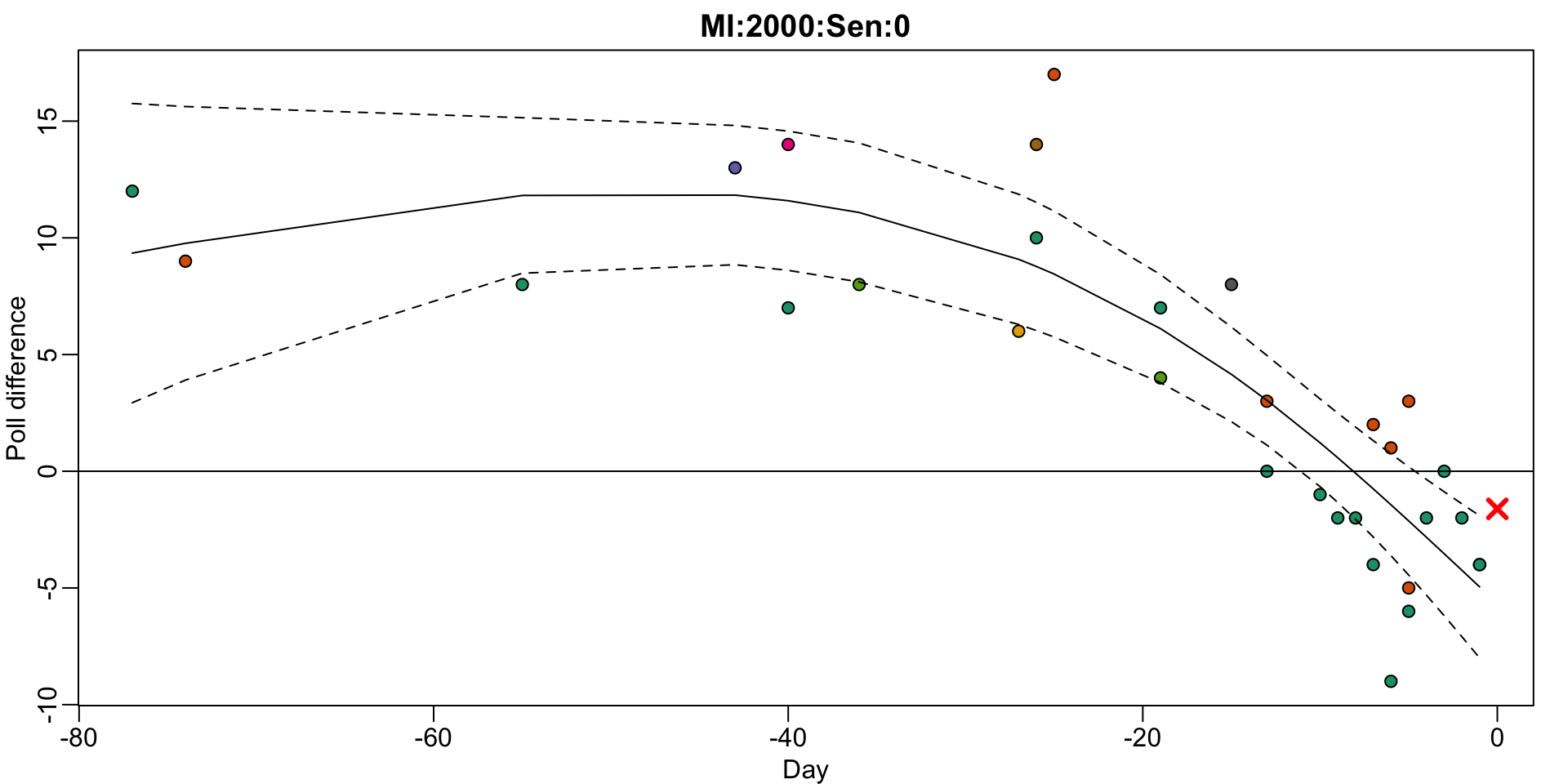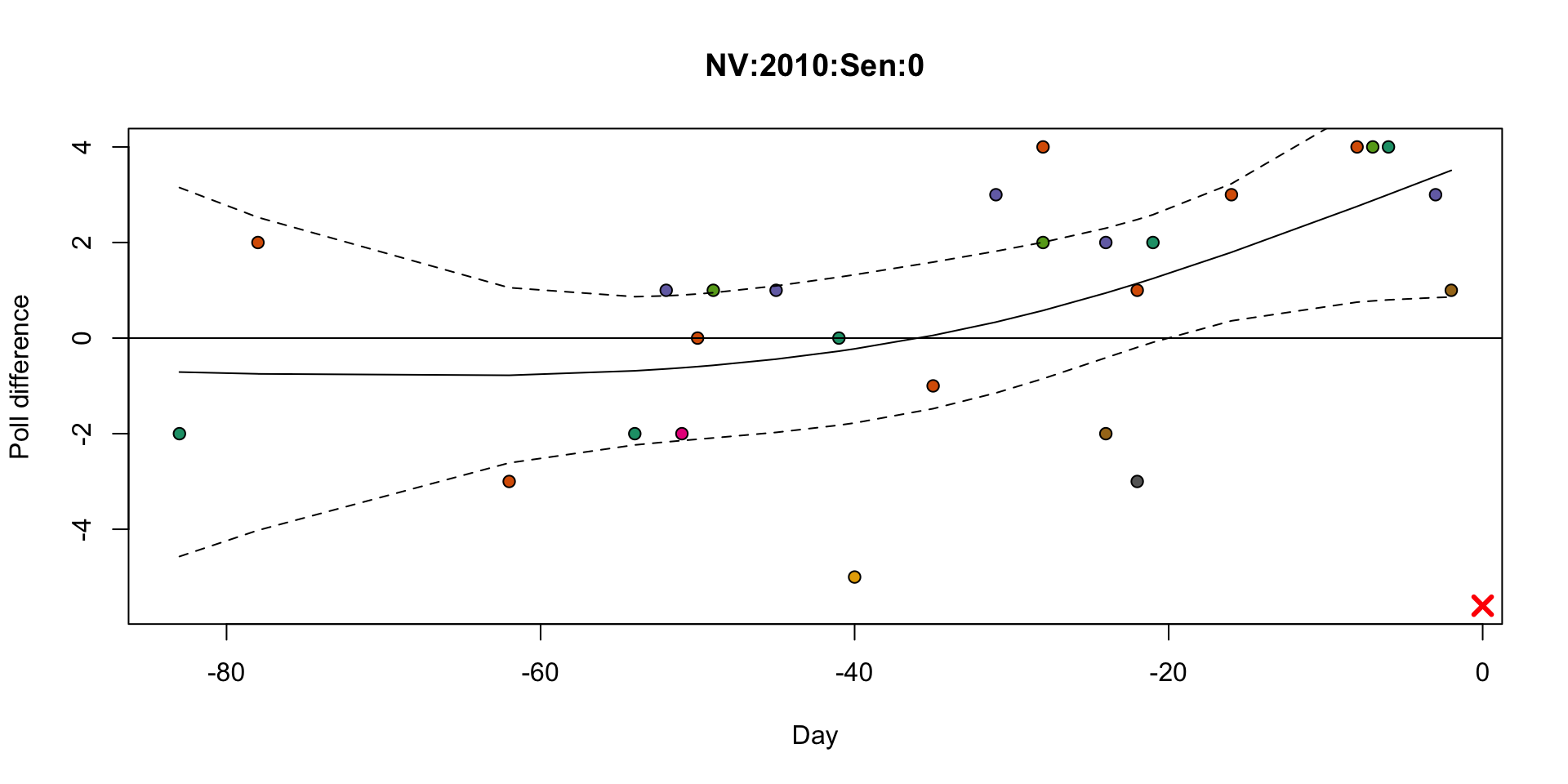Nate Silver does a great job of explaining his forecast model to laypeople. However, as a statistician I’ve always wanted to know more details. After preparing a “predict the midterm elections” homework for my data science class I have a better idea of what is going on. Here is my current best explanation of the model that motivates the way they create a posterior distribution for the election day result. Note: this was written in a couple of hours and may include mistakes.

Let $$\theta$$ represents the real difference between the republican and democrat candidate on election day. The naive approach used by individual pollsters is to obtain poll data and construct a confidence interval. For example by using the normal approximation to the binomial distribution we can write:

$Y = \theta + \varepsilon \mbox{ with } \varepsilon \sim N(0,\sigma^2)$

with $$\sigma^2$$ inversely proportional to the number of people polled. One of the most important insights made by poll aggregators is that this assumption underestimates the variance introduced by pollster effects (also referred to as house effects) as demonstrated by the plot below. For polls occurring within 1 week of the 2010 midterm election this plot shows the difference between individual predictions and the actual outcome of each race stratified by pollster.The model can be augmented to $Y_{i,j} = \theta + h_i + \varepsilon_{i,j} \mbox{ for polls } i=1\dots,M \mbox{ and } j \mbox{ an index representing days left until election}$

Here $$h_i$$ represents random pollster effect. Another important insight is that by averaging these polls the estimator’s variance is reduced and that we can estimate the across pollster variance from data. Note that to estimate $$\theta$$ we need an assumption such as $$\mbox{E}(h_i)=0$$. More on this later. Also note that we can model the pollster specific effects to have different variances. To estimate these we can use previous election. With these in place, we can construct weighted estimates for $$\theta$$ that down-weight bad pollsters.

This model is still insufficient as it ignores another important source of variability: time. In the figure below we see data from the Minnesota 2000 senate race. Note that had we formed a confidence interval, based on aggregated data (different colors represent different pollsters), 20 days before the election we would have been quite certain that the republican was going to win when in fact the democrat won (red X marks the final result). Note that the 99% confidence interval we formed with 20 days before the election was not for $$\theta$$ but for $$\theta$$ plus some day effect.There was a well documented internet feud in which Nate Silver explained why Princeton Election Consortium snapshot predictions were overconfident because they ignored this source of variability. We therefore augment the model to

$Y_{i,j} = \theta + h_i + d_j + \varepsilon_{i,j}$

with $$d_j$$ the day effect. Although we can model this as a fixed effect and estimate it with, for example, loess, this is not that useful for forecasting as we don’t know if the trend will continue. More useful is to model it as a random effect with its variance depending on days left to the election. The plot below, shows the residuals for the Rasmussen pollster, and motivates the need to model a decreasing variance. Note that we also want to assume $$d$$ is an auto-correlated process.If we apply this model to current data we obtain confidence intervals that are generally smaller than those implied by the current 538 forecast. This is because there are general biases that we have not accounted for. Specifically our assumption that $$\mbox{E}(h_i)=0$$ is incorrect. This assumption says that, on average, pollsters are not biased, but this is not the case. Instead we need to add a general bias to the model

$Y_{i,j} = \theta + h_i + d_j + b + \varepsilon_{i,j}.$

But note we can’t estimate $$b$$ from the data: this model is not identifiable. However, we can model $$b$$ as a random effect with and estimate it’s variance from past elections where we know $$\theta$$. Here is a plot of residuals that give us an idea of the values $$b$$ can take. Note that the standard deviation of the yearly average bias is about 2. This means that the SE has a lower bound: even with data from $$\infty$$ polls we should not assume our estimates have SE lower than 2.Here is a specific example where this bias resulted in all polls being wrong. Note where the red X is.Note that, despite these polls predicting a clear victory for Angle, 538 only gave her a 83% of winning. They must be including some extra variance term as our model above does. Also note, that we have written a model for one state. In a model including all states we could include a state-specific $$b$$ as well as a general $$b$$.

Finally, most of the aggregaors report statements that treat $$\theta$$ as random. For example, they report the probability that the republican candidate will win $$\mbox{Pr}(\theta>0 | Y)$$. This implies a prior distribution is set: $$\theta \sim N(\mu,\tau^2)$$. As Nate Silver explained, 538 uses fundamentals to decide $$\mu$$ while $$\tau$$ can be deduced from the weight that fundamentals are given in the light of poll data:

“This works by treating the state fundamentals estimate as equivalent to a “poll” with a weight of 0.35. What does that mean? Our poll weights are designed such that a 600-voter poll from a firm with an average pollster rating gets a weight of 1.00 (on the day of its release55; this weight will decline as the poll ages). Only the lowest-rated pollsters will have a weight as low as 0.35. So the state fundamentals estimate is treated as tantamount to a single bad (though recent) poll. This differs from the presidential model, where the state fundamentals estimate is more reliable and gets a considerably heavier weight.”

I assume they used training/testing approaches to decide on this value of $$\tau$$. But also note that it does not influence the final result of races with many polls. For example, note that for a race with 25 polls, the data receives about 99% of the weight making the posterior practically equivalent to the sampling distribution.

Finally, because the tails of the normal distribution are not fat enough to account for the upsets we occasionally see, 538 uses the Fleishman’s Transformation to increase these probabilities.

We have been discussing these ideas in class and part of the homework was to predict the number of republican senators. Here are few example example. The student that provides the smallest interval that includes the result wins (this explains why some took the risky approach of a one number interval). In a few hours we will know how well they did.